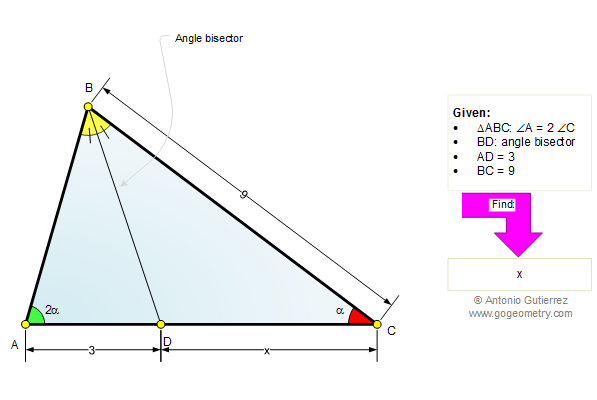Home Geometry Mind Map Problems All Problems 491-500 Double Angle Solution / comment        By Antonio Gutierrez
 Problem 498. Triangle, Angle Bisector, Double Angle, Measure. Level: High School, College, SAT Prep. The drawing shows a triangle ABC with the angle bisector BD. The angle A is double the angle C, AD = 3, and BC = 9. Find CD.Recent Additions